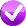# Building Java Programs, 3rd edition

## Lab: While Loops

Except where otherwise noted, the contents of this document are Copyright 2013 Stuart Reges and Marty Stepp.

lab document created by Marty Stepp, Stuart Reges and Whitaker Brand

# Lab goals

Goals for this problem set:

• use `while` loops for indefinite repetition
• practice the fencepost and sentinel loop patterns
• Where you see this icon, you can click it to check the problem in Practice-It!# `while` Loops

A `while` loop repeats indefinitely until a given condition is met.

```while (test) {
statement(s);
}
```

Example:

```int num = 1;
while (num < 5) {
System.out.print(n + " ");     // output: 1 2 3 4
n++;
}
```

# Exercise : `while` loop basics

Consider the following loop.

```int x = 1;
System.out.print(x);
while (x < 100) {
x = x + x;
System.out.print(", " + x);
}
```
 How many times does the code in the `while` loop execute? 7 What output is produced by the overall code? 1, 2, 4, 8, 16, 32, 64, 128

# Exercise : `while` loop mysteryFill in the boxes at right with the output produced by each method call.

```public static void mystery(int x) {
int y = 1;
int z = 0;
while (2 * y <= x) {
y = y * 2;
z++;
}
System.out.println(y + " " + z);
}
```
 `mystery(1);` `1 0` `mystery(6);` `4 2` `mystery(19);` `16 4` `mystery(39);` `32 5` `mystery(74);` `64 6`

# Exercise : `while` loop mysteryFill in the boxes at right with the output produced by each method call.

```public static void mystery2(int x, int y) {
int z = 0;
while (x % y != 0) {
x = x / y;
z++;
System.out.print(x + ", ");
}

System.out.println(z);
}
```
 `mystery2(25, 2);` `12, 1` `mystery2(32, 4);` `0` `mystery2(10345, 10);` `1034, 103, 10, 3` `mystery2(63, 2);` `31, 15, 7, 3, 1, 0, 6`

# Exercise : `while` loop mysteryFill in the boxes at right with the output produced by each method call.

```public static void mystery3(int x) {
int y = 0;
while (x % 2 == 0) {
y++;
x = x / 2;
}
System.out.println(x + " " + y);
}
```
 `mystery3(19);` `19 0` `mystery3(42);` `21 1` `mystery3(48);` `3 4` `mystery3(40);` `5 3` `mystery3(64);` `1 6`

# Exercise : `while` loop mysteryFill in the boxes at right with the output produced by each method call.

```public static void mystery4(int n) {
int x = 1;
int y = 2;
while (y < n) {
if (n % y == 0) {
n = n / y;
x++;
} else {
y++;
}
}
System.out.println(x + " " + n);
}
```
 `mystery4(2);` `1 2` `mystery4(5);` `1 5` `mystery4(24);` `4 3` `mystery4(28);` `3 7`

# Exercise : digitSum• Write a method named `digitSum` that accepts an integer as a parameter and returns the sum of the digits of that number. For example, the call `digitSum(29107)` returns 2+9+1+0+7 or 19. For negative numbers, return the same value that would result if the number were positive. For example, `digitSum(-456)` returns 4+5+6 or 15. The call `digitSum(0)` returns 0.
• (Hint: This is a cumulative algorithm. To extract a digit from a number, `use / 10` and `% 10` operations.)
• Try solving this problem in Practice-It! using the link above.

# Exercise : ProcessName2Modify your previous `ProcessName` program so that it re-prompts until the user types a name that is at least 5 letters total in length and has at least one space in it. Example:

```Type your name: Joe
Error, must be at least 5 chars with a space.
Error, must be at least 5 chars with a space.
Error, must be at least 5 chars with a space.
```
• Try solving this problem in Practice-It! using the link above.

# Fencepost Loops

A fencepost loop is a common algorithmic pattern where you want to perform N tasks with N-1 things between them. It's like a fence with N posts with N-1 wires between the posts.

To achieve this, place one "post" outside your loop, then alternate between "wires" and "posts" inside the loop.

Example:

```System.out.print(1);                 // |==|==|==|==| fence
for (int i = 2; i <= 5; i++) {
System.out.print(".." + i);      // 1..2..3..4..5
}
```

# Exercise : printLetters• Write a method named `printLetters` that takes a `String` as its parameter and that prints the letters of the String, separated by dashes. For example, the call of `printLetters("Rabbit")` should print:
```R-a-b-b-i-t
```
• (Hint: This is a fencepost problem. Remember that each `String` object has a `length` method that tells you how many characters are in the `String` and a `charAt` method that gets you individual characters of the `String`.)
• Try solving this problem in Practice-It! using the link above.

# Exercise : printFactors• Write a method named `printFactors` that accepts an integer as its parameter and uses a fencepost loop to print the factors of that number, separated by the word `"and"`. For example, the call `printFactors(24)` should print the following output:
```1 and 2 and 3 and 4 and 6 and 8 and 12 and 24
```
• You may assume that the parameter value passed is greater than 0.
• (Hint: This is an example of a fencepost problem.)
• Try solving this problem in Practice-It! using the link above.